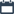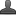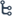## Thursday, October 29, 2020

•October 29, 2020
••, ,
•No comments

## Important questions for 10th class Maths chapter-9 Tangent and Secants to a Circle,Mathematics questionnaire-x class

9.TANGENTS AND SECANTS TO A CIRCLE

Hi friends and my dear students! In this post, I have covered Important questions for 10th class maths chapter-8 Similar triangles, Chapter wise previous year Questions. After Reading  Please do share it with your friends. Learn maths for All classes here

PRIORITY-I

1 MARK QUESTIONS:

1. Define a tangent and a secant to a circle

2. Find the length of a tangent to a circle with centre O and radius 6cm from a point such that OP = 10cm?

3. Draw a circle and two lines parallel to given line such that one is a tangent and the other a secant to the circle?

4. Calculate the length of a tangent from a point 15cm away from the centre of circle of radius 9cm?

5. Find the area of sector whose radius is 7cm, with the given angle 600?

6. The length of the minute hand of a clock is 14cm. Find the area of swept by minute hand in 10minutes?

2 MARKS QUESTIONS

7. Prove that the tangent at any point of a circle is perpendicular to radius through the point of contact?

8. A tangent PQ at a point P of a circle of radius 5cm meets a line through the centre O at a point Q so that OQ= 12cm. find the length of PQ?

9. Prove that the tangents to a circle at the end points of a diameter are parallel?

10. Prove that the lengths of tangents drawn from an external point to a circle are equal?

11. If a circle touches all four sides of a quadrilateral ABCD at points PQRS, then prove that AB+CD= BC+DA?

12. Two concentric circles are radii 5cm, 3cm are drawn. Find the length of the chord of the larger circle which touches the smaller circle?

13. Prove that the parallelogram circumscribing a circle is a rhombus?

14. A chord of circle of a radius 10cm subtends a right angle at the centre. Find the area of the corresponding minor segment and major segment?

15. A chord of circle of a radius 12cm subtends an angle of 1200 at the centre. Find the area of the corresponding minor segment of the circle?

4 MARKS QUESTIONS

16. Draw a pair of tangents to a circle of radius 5cm which are inclined to each other at an angle 600?

17. Draw a circle of radius 6cm. from a point 10cm away from its centre, construct the pair of tangents to the circle and measure their lengths. Verify by using Pythagoras theorem?

18. Construct a tangent to a circle 4cm from a point on the concentric circle of radius 6cm and measure its length. Also verify the measurement by actual calculation?

19. Draw a circle with the help of a bangle. Take a point outside the circle construct the pair of tangents from this point to the circle measure them write conclusion.

20. Find the area of the segment AYB showing in the adjacent figure. If radius of the circle is 21 cm and AOB = 1200?

21. Find the area of the segments shaded in figure, if PQ = 24 CM., PR = 7 CM. and QR is the diameter of the circle with centre O (Take π=22/7)?

Also Check

## Important questions for 10th class Maths chapter-7 Coordinate Geometry

PRIORITY II:

MARK QUESTIONS:

1. ON SIDE 3 AB as diameter of right angled triangle ABC is a circle is drawn intersecting hypotenuse AC in p prove PB=PC

2. Write the formulae for segment area in a circle radius ‘r’ given that the sector angle is ‘𝜽’

3. What is the angle subtended is a circle is centre radius 6cm by an arc of length 3πcm

4. Find the length of tangent drawn from a point 8cm away flows the center of a circle of radius 6cm

5. How many parallel tangents can a circle have?

6. Two concentric circles have center at ‘o’ OP=4cm an OB=5cm AB is a chord of outer circle and a tangent to inner circle at p find length of p

7. AP and AQ are two tangents of a circle with center ‘o’ and  ∟Q=  450 then find  ∟POQ

8. What can you say about the tangents drawn at ends of diameter of a circle?

9. Draw rough sketch of tangent drawn from external point

10. The circumference of a circle exceeds the diameter by 16.8cm find the circumference of the circle

11. What is the difference between a tangent and secant

12. Write the formula for the area of a sector and explain the variables used in the formula

13. How can you mark the centre of a circle if the centre of a circle is unknown?

14. How can you find the area of major circle segment using area of minor circle segment?

15. The tangents to a circle at the end points of diameter are parallel represent the statement diagrammatically

16. Define the segment of a circle? Draw different types of segments of a circle?

17. A point is 13cm away from the center of the circle the length of the tangent drawn from A to the circle is 12cm find the radius of the circle

18. A line through the centre o of a circle of radius 7cm cuts the tangent at a point p on the circle at Q such that PQ=24cm find OQ

2 MARKS QUESTAIONS:

1. AB and AC are two tangents to a circle with centre ‘o’ . if∟OAB OAB =x then find the value of ‘x’ OAB

2. If area of a regular hexagon is 24√3 sq.cm find its side

4. A chord AB of 8cm is drawn is a circle with centre ‘o’ of radius 5cm find the length of tangents from external point p to A and B

5. The length of tangent to a circle of radius 2.5cm from an external point ‘p’ is 6cm find distance of p from nearest point to a circle

6. The area of circle is 220cm2 then find area of square inscribed in it

7. If radius of two concentric circles is 8cm and 10cm then find length of chord of larger circle which is tangent to other

8. A sector is cut from a circle of radius 7cm if the angle of sector is 720 find area

9. If two tangents to a circle of radius 5cm are inscribed to each other at angle of 600 then find the length of each tangents

4 MARKS QUESTIONS:

1. A car has two wipers which do not overlap. Each wiper has a blade of length 25 cm. sweeping through an angle of 115°. Find the total area cleaned at each sweep of the blades.?

2. Find the area of the shaded region in figure, where ABCD is a square of side 10cm. and semicircles are drawn with each side of the square as diameter (use π = 3.14)

3. Find the area of the shaded region in figure, if ABCD is a square of side 7cm. and APD and BPC are semicircles.

4. AB and CD are respectively arcs of two concentric circles of radii 21 cm and 7 cm with centre O. If ∟AOB =300 then find the area of the shaded region(use = π=22/7)

5. A round table top has six equal designs, if the radius of the table top is 14cm then find the cost of making the designs with paint at the rate of 5/- per cm2

6. Calculate the area of the designed region, common between the two quadrants of the circles of radius 10cm each